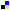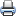# WDM Network DesignSaveDiggDel.icio.usPrint

### Chapter Description

This sample chapter explores some of the design constraints involved in the WDM network design.

## PMD in Long-Haul Design

PMD is not an issue at low bit rates; it becomes a dominant issue at bit rates in excess of 5 Gbps. PMD is inherently caused by the asymmetry of the fiber. This asymmetry adds to a property called birefringence, such that the two principle degenerate modes (polarization modes Ex and Ey) are subject to walkover effect. Due to this walkover effect, the modes are not coupled to each other, which in turn causes the pulse to spread in time.

The main type of PMD considered is second-order PMD, which essentially originates from dispersion due to wavelength dependence of the signal as well as the spectral width of the signal. Figure 4-14 shows the heuristics that create PMD in high bit-rate systems.

A measure of PMD is the differential group delay (DGD), which can simply be visualized as the time difference in multiple spectral components (at multiple speeds) over a given length of fiber. The polarization axes are no longer joint, and the separation increases as the pulse is transmitted through a fiber. The difference is somewhat proportional to the DGD. Therefore, DGD can be accurately used as a measure of PMD for a given system. Moreover, PMD for a given fiber is defined as the mean of DGD. The mean DGD can be calculated from Equation 4-26.

#### Equation 4-26The typical system margin for PMD is 1 dB for general long haul, but it depends on the transmission length.

Consider a numerical example: If the PMD coefficient of the given fiber is 2 ps and the distance under consideration is 625 Km, calculate the DGD. Refer to Equation 4-27.

#### Equation 4-27In 10 G and 40 G systems, a DGD of this magnitude degrades the performance of the system (causes more BER).

Figure 4-15 shows the basic flow diagram that we would use to design an optical network based on system design principles explained so far. One issue that has not been discussed is the tilt limited system, whereby the gain of the amplifier produces different amplification for different channels (and hence different noise levels), generating different values of OSNR for different channels. Compensating tilt is a hard task despite the availability of flat band filters (called gain flattening filters) as well as band equalizers, which try to create uniformity to some extent in the optical working band.Figure 4-15 Flow Diagram of a Generic DWDM Design Case